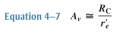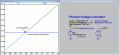# r'e Transistor Internal ac Emitter Resistance

#### SamR

Joined Mar 19, 2019
4,914
Maybe I'm jumping the gun or overthinking this but I am givenOK, nice and simple and was given the derivation of it so I understand how we got there, but where do I find r'e? Not on the datasheets! I did find that Shockley derived it working on diodes and was given that r'e=25mV/IE. Huh? I'm assuming that Google mangles subscripts as this editor does and that it should be I(sub)E? At least V/I conforms to Ohm's law.

In the examples and problems, at least it provides the value but no reference as to its origin. Is it even in common usage and what is the typical source?

#### Papabravo

Joined Feb 24, 2006
20,609
Maybe I'm jumping the gun or overthinking this but I am given
View attachment 272754
OK, nice and simple and was given the derivation of it so I understand how we got there, but where do I find r'e? Not on the datasheets! I did find that Shockley derived it working on diodes and was given that r'e=25mV/IE. Huh? I'm assuming that Google mangles subscripts as this editor does and that it should be I(sub)E? At least V/I conforms to Ohm's law.

In the examples and problems, at least it provides the value but no reference as to its origin. Is it even in common usage and what is the typical source?
It is in fairly common use, but it is a function of junction temperature. The 25mV is actually the value of kT/q for a Kelvin Temperature of 290 °K or 17 °C. As you can see the value is a linear function of temperature. In this expression k is Boltzmann's constant and q is the charge on an electron.It comes from the linearization of the Schockley equation.
https://electronics.stackexchange.c...er-resistance-of-transistor-amplifier-biasing

•DickCappels and SamR

#### LvW

Joined Jun 13, 2013
1,683
Maybe I'm jumping the gun or overthinking this but I am given
View attachment 272754
OK, nice and simple and was given the derivation of it so I understand how we got there, but where do I find r'e? Not on the datasheets! I did find that Shockley derived it working on diodes and was given that r'e=25mV/IE. Huh? I'm assuming that Google mangles subscripts as this editor does and that it should be I(sub)E? At least V/I conforms to Ohm's law.

In the examples and problems, at least it provides the value but no reference as to its origin. Is it even in common usage and what is the typical source?
Yes - that is the problem with this "obscure" quantity rè .
I must admit that I do not like this way of describing the most important property of the BJT - because it defines the voltage gain.

Explanation: The quantity (sometimes erroneously called "intrinsic emitter resistance") is nothing else than the inverse transconductance rè=1/gm.
The transconductance tells you how much the collector current will change as a result of a change in the base-emitter voltage.
It is nothing else than the slope of the chracteristic curve Ic=f(Vbe).
This curve has an exponential form and can easliy be differentiated:
gm=d(Ic)/d(Vbe)=Ic/Vt. (Ic=DC collector current; Vt=Temperature voltage kT/q).

Final comment: The quantity rè is neither a resistance nor belongs it to the emitter - it is transfer quantity with the dimension V/A)

#### SamR

Joined Mar 19, 2019
4,914
OK, thx. Which comes first, adjusting r'e for temp or solving for the xstr and then derating for temp? Since r'e is used for resolving voltage, I would think adjusting/solving it for 25°C would be first?

#### Papabravo

Joined Feb 24, 2006
20,609
OK, thx. Which comes first, adjusting r'e for temp or solving for the xstr and then derating for temp? Since r'e is used for resolving voltage, I would think adjusting/solving it for 25°C would be first?
The temperature to use is not the ambient temperature, but the junction temperature. A transistor running hot and dissipating a moderate amount of heat will have a higher value of r'e.

#### SamR

Joined Mar 19, 2019
4,914
No, but the PDF specs for various values are for 25°C. So, then the general thermal Derating Factor does not affect the r'e which would have to be done in addition to using the Derating Factor. If I understand you correctly? Or simply use A=Vout/Vin, but that would give you the simple answer @ ~room temp. Which is what this book is getting away from to a more detailed answer. Looks like I would need to adjust r'e to get a more exact analysis. K, thx!

As to junction temp. I can measure the device temperature using a thermocouple with my meter, but the actual junction temp is more of a research area determination beyond my ability.

#### LvW

Joined Jun 13, 2013
1,683
Which is what this book is getting away from to a more detailed answer. Looks like I would need to adjust r'e to get a more exact analysis. K, thx!.
In this context (temperature influence) you should realize that the term k*T within the expression for Vt (resp. transconductance gm) plays only a minor role.
The most important influence comes from the saturation current (Is) which appears as a dominating factor in Ic=Is[(exp(Vbe/Vt)-1].
The saturation current Is is very sensitive to temperature variations - and THIS is the reason for some "adjustments" (better: Stabilization against tolerances and variations) - as for example: Negative feedbackprovided by an emitter resistance RE.

•SamR

#### Audioguru again

Joined Oct 21, 2019
6,449
My simulation shows an Re of the transistor= 6.29 ohms at 3.75mA. Then the voltage across the 6.29 ohms is 3.75mA x 6.29 ohms= 23.6mV Which is Wrong!

#### LvW

Joined Jun 13, 2013
1,683
My simulation shows an Re of the transistor= 6.29 ohms at 3.75mA. Then the voltage across the 6.29 ohms is 3.75mA x 6.29 ohms= 23.6mV Which is Wrong!
Why do you write (and calculate with) Re ? This is not a STATIC resistor. It is not a resistor at al!
As I have mentioned earlier - rè is a dynamic (differential) quantity according to rè=1/gm.
Your contribution clearly shows why I do not prefer the writing rè instead of 1/gm. Misinterpretations and misunderstandings are very probable.

Last edited:

#### DickCappels

Joined Aug 21, 2008
10,104
My simulation shows an Re of the transistor= 6.29 ohms at 3.75mA. Then the voltage across the 6.29 ohms is 3.75mA x 6.29 ohms= 23.6mV Which is Wrong!
That formula gives the dynamic resistance - the resistance to infinitesimal changes in voltage, which actually relates Gm of the device. Think about how AGC works in a transistor radio.

#### Audioguru again

Joined Oct 21, 2019
6,449
To reduce distortion, I never used a transistor without an unbypassed emitter resistor so the very small value of the dynamic emitter "resistance" did not matter. Now, opamps are used as amplifiers.

#### LvW

Joined Jun 13, 2013
1,683
To reduce distortion, I never used a transistor without an unbypassed emitter resistor so the very small value of the dynamic emitter "resistance" did not matter. Now, opamps are used as amplifiers.
I think, just the opposite is true.
Look here:
* When the emitter resistor Re is bypassed, the gain of the common-emitter stage is
A=-gmRc=-Rc/rè (using the notation gm=1/rè).

* When the emitter resistor is NOT bypassed, the gain is
Acl=- gmRc/(1+gmRE)=Rc/[(1/gm)+RE]=Rc/[rè + RE].

Therefore, when RE>>rè, the small differential part rè can be neglected.
Hence, such a simplification may be allowed only when we have negative signal feedback.

I think, your contribution is a classical example for the danger of misinterpretation of the quantity rè. It is NOT an "emitter resistance". It is just the inverse of the transconductance.

Last edited:
•ericgibbs